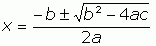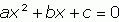SEARCH HOMEMath Central Quandaries & QueriesQuestion from Stuart, a student: Solve the following quadratic equation: 3xsquared-5x-4=0Hi Stuart.

There is a special formula called the Quadratic Formula which every student should memorize:To use this formula, you need to determine the values of a, b and c by putting your equation in the form:(which yours already is). So for you, a = 3, b = -5 and c = -4. Put these values into the Quadratic Formula and you will find your solution(s).

Note: when you use the quadratic formula (which you can always use in place of factoring if you like), you usually find two solutions because of the ± in there. But if the value of b2 - 4ac is zero, you get only one solution and if the value of b2 - 4ac is negative, then there is no real solution at all.

Hope this helps,
Stephen La Rocque.Math Central is supported by the University of Regina and The Pacific Institute for the Mathematical Sciences.